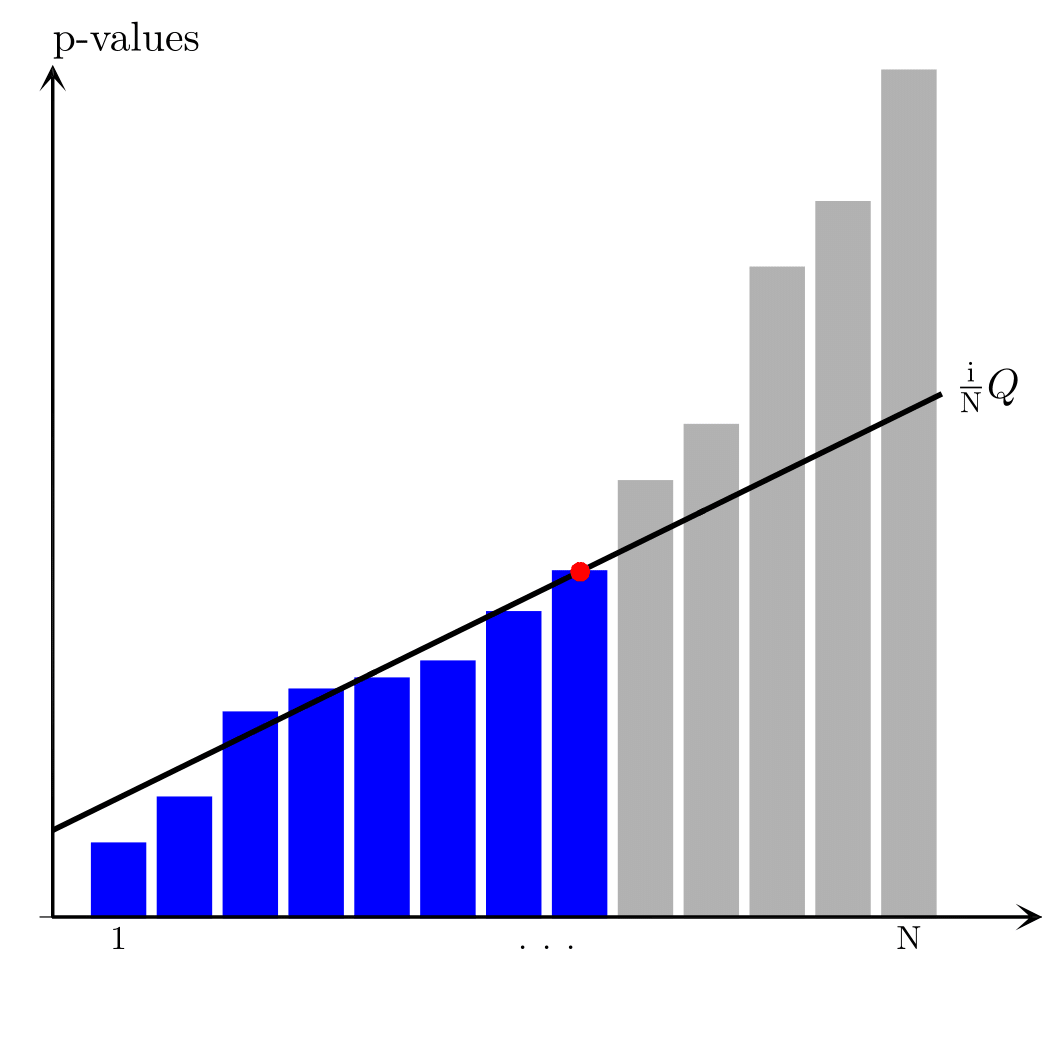# fdr-eps-converted-to-1The individual p-values for each hypothesis testing are arranged in order starting from the smallest one and compared to their false-rate-corrected values calculated as ${i \over N}Q$ (here, $Q=0.10$). The largest p-value that is smaller than the corresponding false-rate-corrected value is significant as well as all hypothesis having smaller p-values (regardless of whether they are larger than the corresponding false rate corrected values).